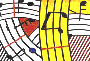Tutorial 22 - Chord-seq I :

Onsets and durations I

Topics

Managing durations in the CHORD-SEQ editor.

Functions used

CHORD-SEQ
,dx->x, x-dx

Description

Usually, in traditional music notation rhythm is represented as a single value (example, quarter note, eight notes, etc...). This is rather a simplification of what commonly is understood by rhythm. In fact, the rhythmical value alone is not a sufficient information in order to place notes in time. One also needs to know, when and where (in time) these values will appear. That is where metric information is required. In the same manner, in Openmusic and most computer musical environments, rhythm and durations are represented through two values:

- Onsets are the date of notes giving the "time address" of each event.

- Durs are the durations of these events.

Both will be notated in millliseconds.

Now let us consider the example below: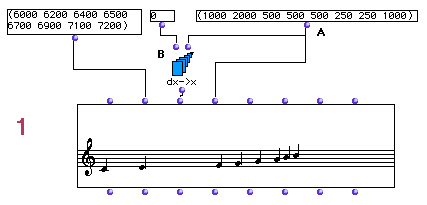Patch structure
A: Assuming a given list of durations in milliseconds

(1000 2000 500 500 500 250 250 1000)

which is equivalent to: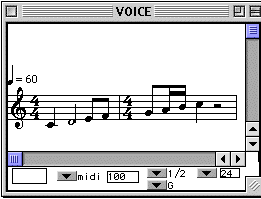we will connect it to the fourth input of CHORD-SEQ .

B: In order to have the correct onsets for this list we will use dx->x method which will output

(0 1000 3000 3500 4000 4500 4750 5000 6000)

Notice that the first input of dx->x is 0 meaning that our seqence starts at zero time (offset).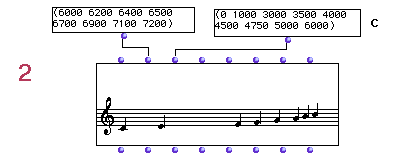In example 2 we will use the same list of onsets (C). This will result in an erronous duration values, i.e all duration being at a default value of 1000 milliseconds the sequence will have rests or overlaping notes as it is shown below.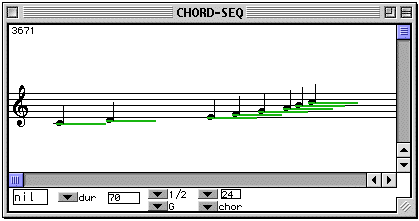In order to avoid this we will use the eigth input of CHORD-SEQ (legato) set to 100 (D)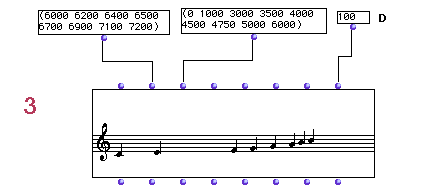In this case all durations will be set automatically to the correct intervals between onsets and therefore computing our initial duration list as in example 1.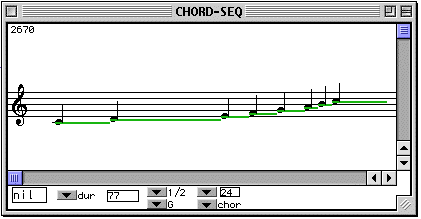This will be the same as in using the inverse operation of dx->x which is x->dx. ( Notice that we have already used in tutorial 11 and tutorial 14 the legato set to 100).